# GMAT Math : Circles

## Example Questions

### Example Question #26 : Dsq: Calculating The Length Of A Radius

Rectangle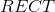is inscribed inside a circle. What is the radius of the circle?

Statement 1: Rectanglehas area 200.

Statement 2: Rectanglehas perimeter 60.

Statement 2 ALONE is sufficient to answer the question, but Statement 1 ALONE is NOT sufficient to answer the question.

EITHER statement ALONE is sufficient to answer the question.

BOTH statements TOGETHER are sufficient to answer the question, but NEITHER statement ALONE is sufficient to answer the question.

Statement 1 ALONE is sufficient to answer the question, but Statement 2 ALONE is NOT sufficient to answer the question.

BOTH statements TOGETHER are insufficient to answer the question.

BOTH statements TOGETHER are sufficient to answer the question, but NEITHER statement ALONE is sufficient to answer the question.

Explanation:

The diameter of a circle with an inscribed rectangle is equal to the length of a diagonal of the rectangle, which, given the lengthand width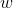, can be found using the Pythagorean Theorem: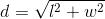Statement 1 alone gives insufficient information. For example, a 20 by 10 rectangle has area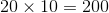, and a 40 by 5 rectangle has area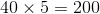The first rectangle has diagonals of length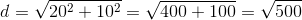.

The second rectangle has diagonals of length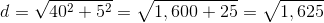,

Since the diagonals of the rectangles differ, so do the diameters, and, consequently, the radii, of the circles.

Statement 2 alone gives insufficient information for a similar reason. For example, a 20 by 10 rectangle has perimeter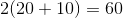, and a 25 by 5 rectangle has perimeter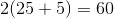. Again, the first rectangle has  diagonals of length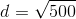. The second has diagonals of length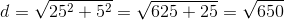.

Now, assume both statements to be true. We are looking for two numbers whose product is 200 and whose sum is 30 (since the perimeter is twice the sum, or 60). The only such pair of numbers can be found by trial and error to be 20 and 10, so these are the length and width of the rectangle. As shown before, a rectangle with these dimensions has diagonals of length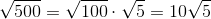. This is the diameter of the circle in which it is inscribed, so half this, or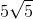, is the radius.

### Example Question #27 : Dsq: Calculating The Length Of A Radius

Give the radius of a circle on the coordinate plane.

Statement 1: Of the three intercepts of the circle, exactly two are-intercepts, one of which is at the point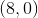.

Statement 2: Of the three intercepts of the circle, exactly two are-intercepts, one of which is at the point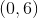.

Statement 2 ALONE is sufficient to answer the question, but Statement 1 ALONE is NOT sufficient to answer the question.

BOTH statements TOGETHER are insufficient to answer the question.

Statement 1 ALONE is sufficient to answer the question, but Statement 2 ALONE is NOT sufficient to answer the question.

EITHER statement ALONE is sufficient to answer the question.

BOTH statements TOGETHER are sufficient to answer the question, but NEITHER statement ALONE is sufficient to answer the question.

BOTH statements TOGETHER are sufficient to answer the question, but NEITHER statement ALONE is sufficient to answer the question.

Explanation:

Statement 1 alone only gives one point through which the circle passes, so no information can be determined about the other points or about the size or location of the circle. A similar argument holds for the insufficiency of Statement 2.

Now assume both statements are true. The circle has exactly three intercepts, but it is given that there are two-intercepts -and one other point - and two-intercepts -and one other point. The unidentified-intercept and the unidentified-intercept must be one and the same, and the only possible way this can happen is for this common point to be the origin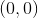. Since three points define a circle, we can now identify the unique circle through the points, and, and we can figure out its radius.

### Example Question #31 : Circles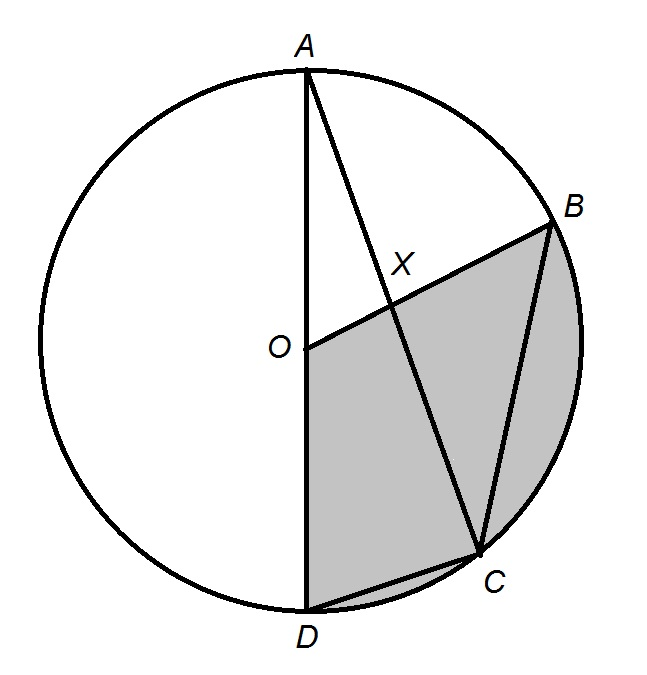Give the radius of the above circle with center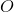.

Statement 1: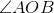is aangle.

Statement 2: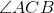is aangle.

EITHER statement ALONE is sufficient to answer the question.

Statement 2 ALONE is sufficient to answer the question, but Statement 1 ALONE is NOT sufficient to answer the question.

Statement 1 ALONE is sufficient to answer the question, but Statement 2 ALONE is NOT sufficient to answer the question.

BOTH statements TOGETHER are insufficient to answer the question.

BOTH statements TOGETHER are sufficient to answer the question, but NEITHER statement ALONE is sufficient to answer the question.

BOTH statements TOGETHER are insufficient to answer the question.

Explanation:

The two statements together only give information about angle measures; arc degree measures can be deduced from this information but not any arc lengths or side lengths. Without this information, we cannot obtain the radius of this circle.

### Example Question #1 : Dsq: Calculating Circumference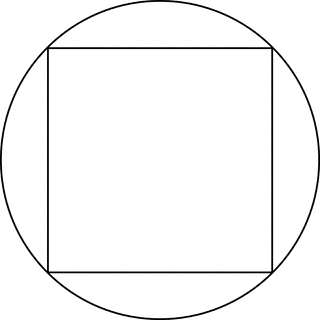Square S is inscribed in circle C as in the figure above. What is the circumference of C?

(1) The perimeter of S is 16.

(2) The area of S is 36.

B. Statement (2) ALONE is sufficient, but statement (1) alone is not sufficient.

A. Statement (1) ALONE is sufficient, but statement (2) alone is not sufficient.

E. Statements (1) and (2) TOGETHER are NOT sufficient.

D. EACH statement ALONE is sufficient.

C. BOTH statements TOGETHER are sufficient, but NEITHER statement ALONE is sufficient.

D. EACH statement ALONE is sufficient.

Explanation:

From statement (1), we know that the side of S is 4, and then we can calculate the diagonal of S using the Pythagorean theorem: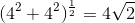. The diagonal of S is the diameter of C. Therefore, we can calculate the circumference by using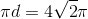. From statement (2), we know that the side of S is 6, and then we can calculate the diagonal of S using the Pythagorean theorem: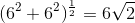The diagonal of S is the diameter of C. Therefore, we can calculate the circumference by using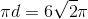.

### Example Question #31 : Radius

What is the circumference of circle J?

I) Circle J has an area of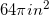.

II) Circle J has a diameter of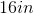.

Statement 2 is sufficient to solve the question, but statement 1 is not sufficient to solve the question.

Statement 1 is sufficient to solve the question, but statement 2 is not sufficient to solve the question.

Each statement alone is enough to solve the question.

Neither statement is sufficient to solve the question. More information is needed.

Both statements taken together are sufficient to solve the question.

Each statement alone is enough to solve the question.

Explanation:

We are given the area and diameter of a circle and asked to find the circumference. We know that diameter is twice the length of a radius, so we also have our radius.

Given the following equations: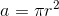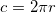We can see that knowing either diameter or area will allow us to find the circumference.

Thus: Each statement alone is enough to solve the question.

### Example Question #1 : Dsq: Calculating Circumference

What is the circumference of the circle given by: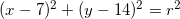I)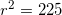.

II) The slope of the tangent to the circle at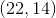is undefined.

Statement II is sufficient to answer the question, but Statement I is not sufficient to answer the question.

Statement I is sufficient to answer the question, but Statement II is not sufficient to answer the question.

Both statements together are needed to answer the question.

Either statement alone is sufficient to answer the question.

Either statement alone is sufficient to answer the question.

Explanation:

All we need to find circumference is the radius.

I) Gives us the radius squared, so we could find circumference with I.

II) Tells us the slope of the tangent line at a given point is undefined. Only vertical lines have undefined slope. The tangent line is perpendicular to the radius, so we can find our radius by drawing a picture and comparing the location of the center to the location of the tangent line.

So either statement will be sufficient.

### Example Question #1 : Dsq: Calculating Circumference

What is the circumference of Circle?

1.) The radius of the circle is.

2.) The circle is inside another circle of area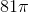.

Statement 1 is sufficient to solve the question, but Statement 2 is not sufficient to solve the question.

Both statements taken together are sufficient to solve the question.

Neither statement is sufficient to solve the question. More information is needed.

Each statement alone is sufficient to solve the question.

Statement 2 is sufficient to solve the question, but Statement 1 is not sufficient to solve the question.

Statement 1 is sufficient to solve the question, but Statement 2 is not sufficient to solve the question.

Explanation:

We are asked to find the circumferenceof Circleand are given the diameter and the area. We also know that.  Taking each statement individually:

1.) The radiusisand we know that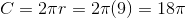. Therefore, Statement 1 is sufficient to solve for the circumference of the circle by itself.

2.) The areaof the outside circle is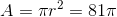, but we cannot use this to determine the circumference of Circlebecause we don't know whereis inside the larger outside circle.

### Example Question #581 : Data Sufficiency Questions

What is the circumference of Circle?

1.) The diameter of the circle is.

2.) The area of the circle is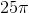.

Statement 2 is sufficient to solve the question, but Statement 1 is not sufficient to solve the question.

Statement 1 is sufficient to solve the question, but Statement 2 is not sufficient to solve the question.

Both statements taken together are sufficient to solve the question.

Neither statement is sufficient to solve the question. More information is needed.

Each statement alone is sufficient to solve the question.

Each statement alone is sufficient to solve the question.

Explanation:

We are asked to find the circumferenceof Circleand are given the diameter and the area. We also know that.  Taking each statement individually:

1.) The diameterisand we know that the radius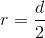, so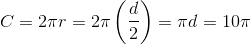. Therefore, Statement 1 is sufficient to solve for the circumference of the circle by itself.

2.) The areaof Circleis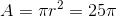, so we can determine that the radius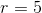. Since the circumference, Statement 2 is is sufficient to solve for the circumference of the circle by itself.

### Example Question #1 : Sectors

Brendan's girlfriend made him a cheescake for his birthday. He eats one slice a day. What is the measure of the central angle of each slice?

I) The diameter of the cake is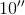.

II) Each slice is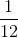of the total cake.

Statement II is sufficient to answer the question, but Statement I is not sufficient to answer the question.

Either statement alone is sufficient to answer the question.

Statement I is sufficient to answer the question, but Statement II is not sufficient to answer the question.

Both statements together are needed to answer the question.

Statement II is sufficient to answer the question, but Statement I is not sufficient to answer the question.

Explanation:

In this case we are given a circle and asked to find the angle of a portion of it.

The diameter would allow us to find many things related to the circle, but not an individual slice.

However, knowing that each slice is 1/12 of the total allows us to multiply 360 by 1/12 and find out that each slice is 30 degrees.

Therefore statement II alone is sufficient in answering the question.

### Example Question #2 : Sectors

One slice of a pizza is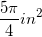. What is the central angle of one slice?

I) Each slice isof the whole pizza.

II) Each straight edge the slice is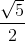inches.

Both statements together are needed to answer the question.

Statement II is sufficient to answer the question, but Statement I is not sufficient to answer the question.

Statement I is sufficient to answer the question, but Statement II is not sufficient to answer the question.

Either statement alone is sufficient to answer the question.